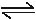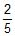Courses

# MCQ (Previous Year Questions) - Ionic Equilibrium (Level 2)

## 16 Questions MCQ Test Chemistry for JEE Advanced | MCQ (Previous Year Questions) - Ionic Equilibrium (Level 2)

Description
This mock test of MCQ (Previous Year Questions) - Ionic Equilibrium (Level 2) for JEE helps you for every JEE entrance exam. This contains 16 Multiple Choice Questions for JEE MCQ (Previous Year Questions) - Ionic Equilibrium (Level 2) (mcq) to study with solutions a complete question bank. The solved questions answers in this MCQ (Previous Year Questions) - Ionic Equilibrium (Level 2) quiz give you a good mix of easy questions and tough questions. JEE students definitely take this MCQ (Previous Year Questions) - Ionic Equilibrium (Level 2) exercise for a better result in the exam. You can find other MCQ (Previous Year Questions) - Ionic Equilibrium (Level 2) extra questions, long questions & short questions for JEE on EduRev as well by searching above.
QUESTION: 1

### The degree of dissociation of water at 25°C is 2 × 10-7 % and density is 1.0 g cm-3. The ionization constant for water is - [IIT-1995]

Solution:
*Multiple options can be correct
QUESTION: 2

### A buffer solution can be prepared from a mixture of -        [IIT-1999]

Solution:
*Multiple options can be correct
QUESTION: 3

### The pH of 0.1 M solution of the following salts increases in the order      [JEE 1999]

Solution: NaCN is prepared from strong Base and weak acid therefore it's ph will be very high after that NaCl is made up of strong acid as well as strong Base so it's ph will be less than NaCN after that NH4Cl is from weak Base and strong acid so it'll be less in ph than NaCl but more than HCl which is a strong acid
*Multiple options can be correct
QUESTION: 4

An aqueous soltion of 6.3 g oxalic acid dihydrate is made up to 250 mL. The volume of 0.1 N NaoH required to completely neutralise 10 mL of this solution is :    [JEE 2001]

Solution:
QUESTION: 5

For sparingly soluble salt ApBq, the relationship of its solubility product (Ls) with its solubility (S) is [JEE 2001]

Solution:
*Multiple options can be correct
QUESTION: 6

A solution which is 10-3 M each in Mn2+, Fe2+, Zn2+ and Hg2+ is treated with 10-16 M sulphide ion. If Ksp, MnS, FeS, ZnS and HgS are 10-15, 10-23, 10-20 and 10-54 respectively, which one will precipitate first ?     [JEE 2003]

Solution:
*Multiple options can be correct
QUESTION: 7

HX is a weak acid (Ka = 10-5). It forms a salt NaX (0.1 M) on reacting with caustic soda. The degree of hydrolysis of NaX is         [JEE 2004]

Solution:
*Multiple options can be correct
QUESTION: 8

CH3NH2 (0.1 mole, Kb = 5 × 10-4) is added to 0.08 moles of HCl and the solution is diluted to one litre, resulting hydrogen ion concentration is      [JEE 2005]

Solution:
*Multiple options can be correct
QUESTION: 9

Ag+ + NH3[Ag(NH3)]+ ; k1 = 3.5 × 10-3

[Ag(NH3)]+ + NH3[Ag(NH3)2]+ ;

k2 = 1.7 × 10-3

then the formation constant of [Ag(NH3)2]+ is                          [JEE 2006]

Solution:
*Multiple options can be correct
QUESTION: 10

The species present in solution when CO2 is dissolved in water:              [JEE 2006]

Solution:
*Multiple options can be correct
QUESTION: 11

Solubility product constant (Ksp) of salts of types MX, MX2 and M3X at temperature 'T' are 4.0 × 10-8, 3.2 × 10-14 and 2.7 × 10-15, respectively. Solubilities (mol. dm-3) of the salts at temperature 'T' are in the order

[JEE 2008]

Solution:
*Multiple options can be correct
QUESTION: 12

2.5 mL ofM weak monoacidic base (Kb = 1 × 10-12 at 25°C) is titrated withM HCl in water at 25°C. The concentration of H+ at equivalence point is (Kw = 1 × 10-4 at 25°C)          [JEE 2008]

Solution:
*Multiple options can be correct
QUESTION: 13

Aqueous solutions of HNO3, KOH, CH3COOH, and CH3COONa of identical cocentrations are provided. The pair(s) of solutions which form a buffer upon mixing is(are).              [JEE 2010]

Solution:

A buffer solution consists of weak acid and its salt with strong base or weak base and its salt with strong acid.
If we limit the amount of nitric acid, then it will partially convert sodium acetate to acetic acid.
CH3COONa+HNO3​→CH3​COOH+NaNO3
Now the solution contains acetic acid (weak acid) and sodium acetate (salt with strong base NaOH). So, it will become a buffer solution.
Acetic acid (weak acid) and sodium acetate (salt with strong base NaOH) also form a buffer solution.
Hence both C and D are correct.

*Multiple options can be correct
QUESTION: 14

The Ksp of Ag2CrO4 is 1.1 × 10-12 at 298 K. The solubility (in mol/L) of Ag2CrO4 in a 0.1 M AgNO3 solution is        [IIT-2013]

Solution:
*Multiple options can be correct
QUESTION: 15

The initial rate of hydrolysis of methyl acetate (1M) by a weak acid (HA, 1M) is 1/100th of a strong acid (HX, 1M) at 25ºC. The Ka of HA is            [IIT-2013]

Solution:
*Multiple options can be correct
QUESTION: 16

The Ksp of Ag2CrO4 is 1.1 × 10-12 at 298 K. The solubility (in mol/L) of Ag2CrO4 in a 0.1 M AgNO3 solution is

Solution: